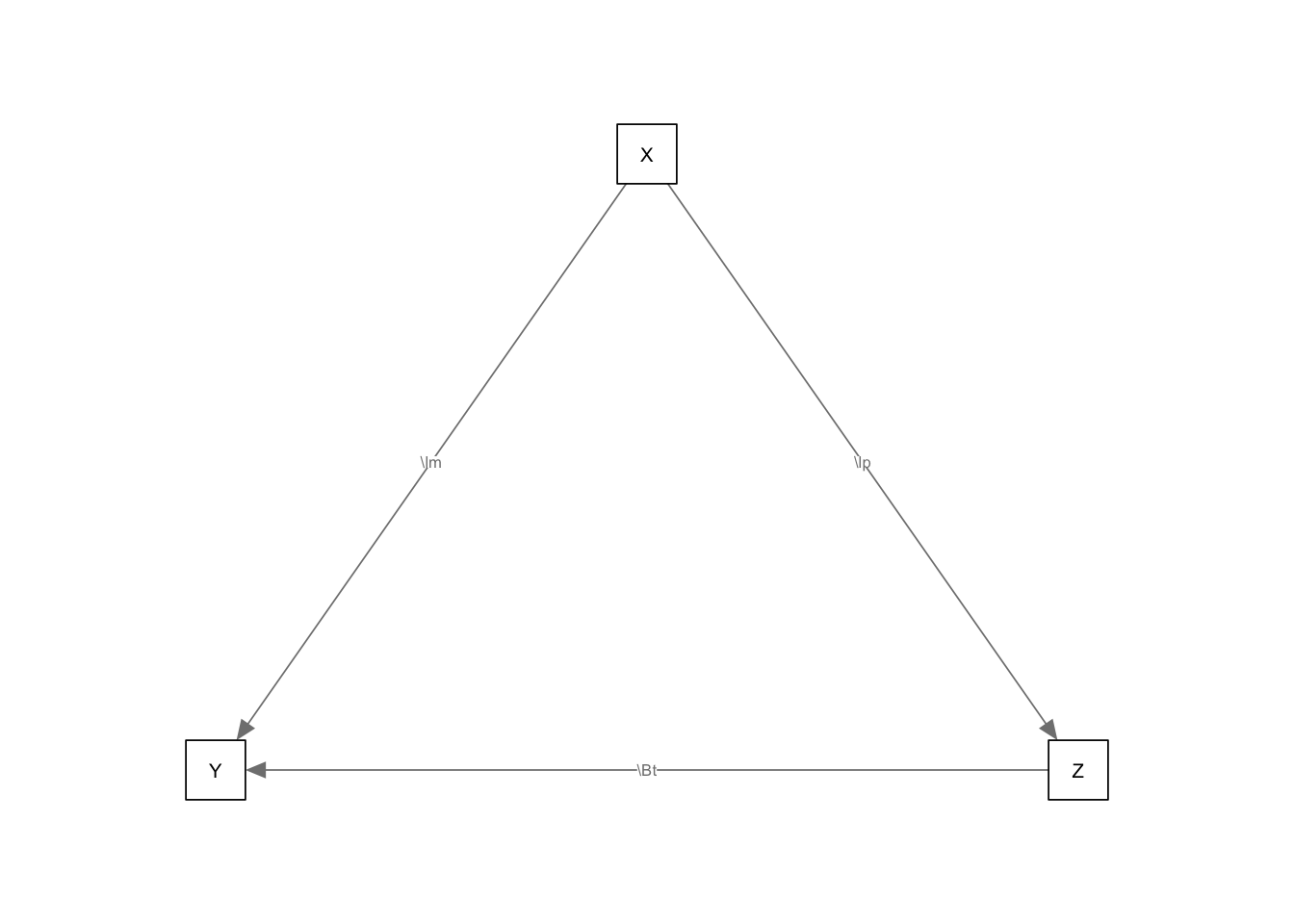In this vignette, we lay out a very simplified mediation model as a means of understanding how Matrices of Implied Causality (MICs) can be applied. We first need to load in the MICr package. If semPlot is installed, we’ll use it, too.

library(MICr)
library(OpenMx)
if(library(semPlot, logical.return=TRUE))  drawPlots<-TRUE else drawPlots <- FALSE 

The primary model is a fairly straightforward mediation model. X is the predictor, Z the mediator, and Y is the outcome.

XYZmodel <- mxModel("XYZ", manifestVars=c("X", "Y", "Z"), type="RAM",
mxPath(from="Z", to="Y", values=.6, labels="\\Beta"),
mxPath(from="X", to=c("Y", "Z"), values=c(.2, .8), labels=c("\\lambda", "\\alpha")),
mxMatrix("Iden",3,name="I"))
if(drawPlots) semPaths(XYZmodel)The model’s MIC looks like this:

MIC(XYZmodel)
##      X Y   Z
## X 1.00 0 0.0
## Y 0.68 1 0.6
## Z 0.80 0 1.0

Note that Z->Y and X->Z are identical to the path loadings, since there is only one path present to follow each link. However, X->Y is two paths: one direct and one indirect. The MIC path between them is the total effect–the combination of the influences across the two paths.

For ease of reporting, this can also be displayed as a MIC table.

MICTable(XYZmodel, caption="Simple Mediation MIC Table")
Simple Mediation MIC Table
from to XYZ
X Z 0.80
X Y 0.68
Z Y 0.60

The table format has the easy ability to select using traditional data frame selection operators, but the MICTable function also has helpers. For example, if we only care about the causal influences on Z or on Y:

MICTable(XYZmodel, caption="Simple Mediation MIC Table: Effects on Y", to="Y")
Simple Mediation MIC Table: Effects on Y
from to XYZ
X Y 0.68
Z Y 0.60
MICTable(XYZmodel, caption="Simple Mediation MIC Table: Effects on Z", to="Z")
Simple Mediation MIC Table: Effects on Z
from to XYZ
X Z 0.8

This allows us to identify the unique elements of the causal structure in a more direct and sensible way.